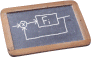# Identification, Analysis, and Control of Dynamical Systems

COURSE DESCRIPTION

Abstract

The course provides an introduction to dynamical systems, with emphasis on linear systems in state-space form. After introducing the basic concepts of stability, controllability and observability, the course covers the main techniques for the synthesis of stabilizing controllers (state-feedback controllers and linear quadratic regulators) and of state estimators (Luenberger observer and Kalman filter). The course also briefly covers data-driven approaches of parametric identification to obtain models of dynamical systems from a set of data, with emphasis on the analysis of the robustness of the estimated models w.r.t. noise on data and on the numerical implementation of the algorithms.

Syllabus

Equilibrium points and stability. Linearization of nonlinear systems. Discretization of continous-time systems. Transfer functions. Observability and controllability of LTI systems. Luenberger's observer and state-feedback controllers. Linear quadratic regulator and Kalman filter. Basic concepts on linear parameter-varying systems. System identification: Least-squares methods and recursive identification, instrumental-variables methods, consistent and unbiased estimators, prediction error methods.

PREREQUISITES

Linear algebra and matrix computation, calculus and mathematical analysis.

LECTURE SLIDES
Last update: February 3, 2020